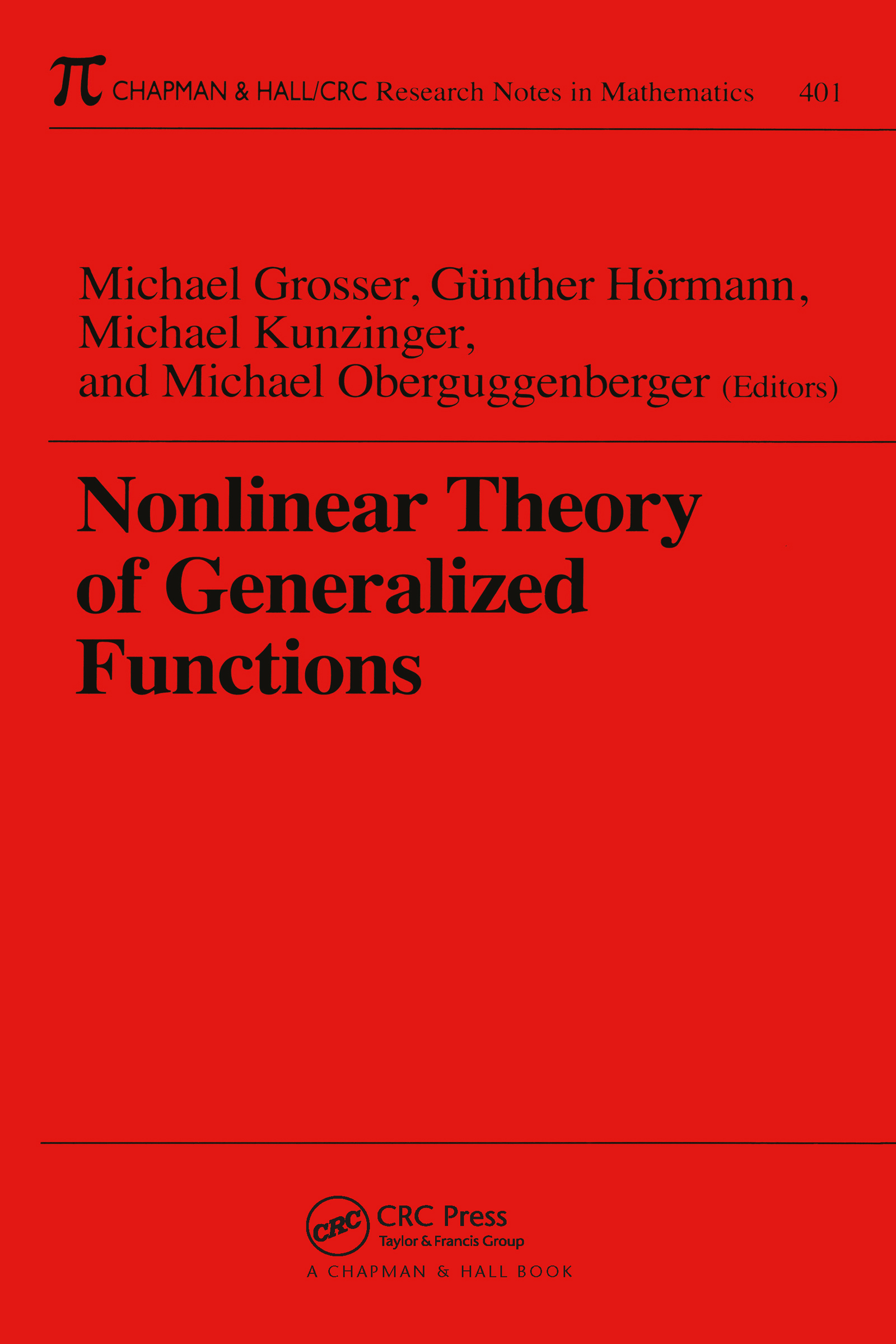# Nonlinear Theory of Generalized Functions

## 1st Edition

Chapman and Hall/CRC

400 pages

##### Purchasing Options:\$ = USD
Paperback: 9780849306495
pub: 1999-03-16
SAVE ~\$35.00
Currently out of stock
\$175.00
\$140.00
x

FREE Standard Shipping!

### Description

Questions regarding the interplay of nonlinearity and the creation and propagation of singularities arise in a variety of fields-including nonlinear partial differential equations, noise-driven stochastic partial differential equations, general relativity, and geometry with singularities. A workshop held at the Erwin-Schrödinger International Institute for Mathematical Physics in Vienna investigated these questions and culminated in this volume of invited papers from experts in the fields of nonlinear partial differential equations, structure theory of generalized functions, geometry and general relativity, stochastic partial differential equations, and nonstandard analysis.

The authors provide the latest research relevant to work in partial differential equations, mathematical physics, and nonlinear analysis. With a focus on applications, this books provides a compilation of recent approaches to the problem of singularities in nonlinear models. The theory of differential algebras of generalized functions serves as the central theme of the project, along with its interrelations with classical methods.

Preface

Partial Differential Equations

On the Structure of Singularities in Solutions of the Nonlinear Schrödinger Equation for the Critical Case, p = 4/n , J. Angulo, J. L. Bona, F. Linares, M. Scialom

The Schrödinger Equation with Point Interaction in an Algebra of New Generalized Functions, A. Antonevich

Polynomial a Priori Estimates for Some Evolution PDE and Generalized Solutions, H. A. Biagioni, T. Gramchev

Shift Differentials of Maps in BV Spaces, A. Bressan, M. Lewicka

Calculation of the Singularity Dynamics for Quadratic Nonlinear Hyperbolic Equations. Example: The Hopf Equation, V. G. Danilov, G. A. Omel'yanov

Vanishing Viscosity Boundary Layers for Nonlinear Hyperbolic Systems, O. Guès

Ordinary Differential Equations and Generalized Functions, R. Hermann, M. Oberguggenberger

Conservation Laws, Delta Shocks and Singular Shocks, B. L. Keyfitz

Nonlinear Singular Schrödinger Type Equations, H. Lange, m. Poppenberg, H. Teismann

Non-Analytic Solutions of Nonlinear Wave Models, Y. A. Li, P. J. Olver, P. Rosenau

The Dirichlet Problem and Compact Operators in Colombeau Theory, D. Scarpalezos

Highly Oscillatory Shock Waves, Y. Wang

Structure Theory

Sharp Topologies on (C, E, P)-Algebras, A. Delcroix, D. Scarpalezos

(C, E, P)-Sheaf Structures and Applications, J.-A. Marti

Local and Microlocal Analysis in the Space of Colombeau Generalized Functions, S. Pilipovic

Basics of a General Spectral Theory of Banach Modules, N. Y. Radyno

Extensions of Algebras, Memofunctions and Their Applications, Y. V. Radyno

On the Multiplication of Periodic Hyperfunctions of One Variable, V. Valmorin

Geometry, General Relativity

Distributional Aspects of General Relativity: The Example of the Energy-Momentum Tensor of the Extended Kerr-Geometry, H. Balasin

Lie Symmetries of Differential Equations in a Generalized Functions Setting, M. Kunzinger

Arbitrary Global Lie Group Actions on Generalized Solutions of Nonlinear PDEs and an Answer to Hilbert's Fifth Problem, E. E. Rosinger

Distributional Description of Impulsive Gravitational Waves, R. Steinbauer

Non-Linear Generalized Functions in General Relativity, J. A. Vickers

Stochastic Analysis

A White Noise Approach to Stochastic Differential Equations Driven by Wiener and Poisson Processes, H. Holden, B. Øksendal

White Noise Driven Stochastic Partial Differential Equations: Triviality and Non-Triviality, F. Russo, M. Oberguggenberger

Measurement Methods Related to Differential Equations, J. Ubøe

On the Small Time Asymptotics of Solutions of Linear and Non-Linear Stochastic Differential Equations, T. Zhang

Nonstandard Methods

The Global Control of Shock Waves, J.E. Rubio

Pointwise Values and Fundamental Theorem in the Algebra of Asymptotic Functions, T. D. Todorov

### Subject Categories

##### BISAC Subject Codes/Headings:
MAT003000
MATHEMATICS / Applied
MAT007000
MATHEMATICS / Differential Equations